# Instructions for using the Rank function in Excel to arrange rankings simply

While making reports or statistics of products, quantities, etc. in Excel, you need to perform ranking division. Doing ranking in Excel will become simpler than ever thanks to the Rank function in Excel. In the following article, we will guide you to use the Rank function in Excel easily and quickly in just one step.## What is the Rank function in Excel?

The Rank function in Excel is a function that uses the ranking of data or graphs. When using this function, you can easily sort and rank data in order from high to low or low to high. The Rank function has a relatively similar task to when we perform data sorting, but it is more superior and more optimal.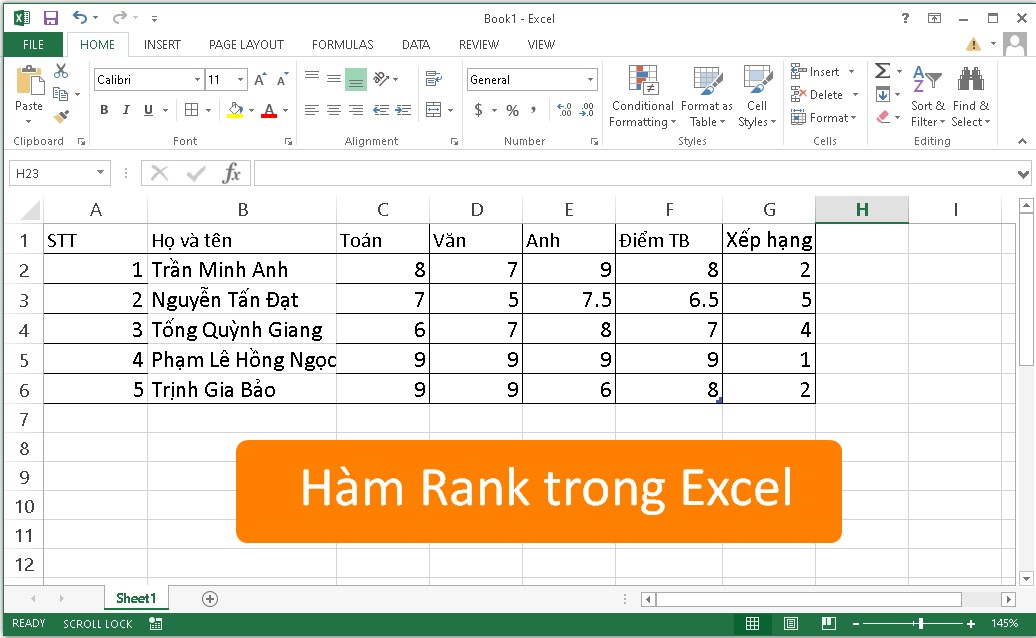### In which case is the Rank function used?

• The Rank function you can use to sort the data in order from highest to lowest.
• Or when you want to sort the ranking of the data from low to high.

## Instructions for using the Rank function in Excel to rank

### Rank Excel function formula

With the formula of the Rank function, you can perform a check on a certain number or you can know what the rank of that position in the table is. Use the following formula:

Formula Rank=RANK(number,ref, [order])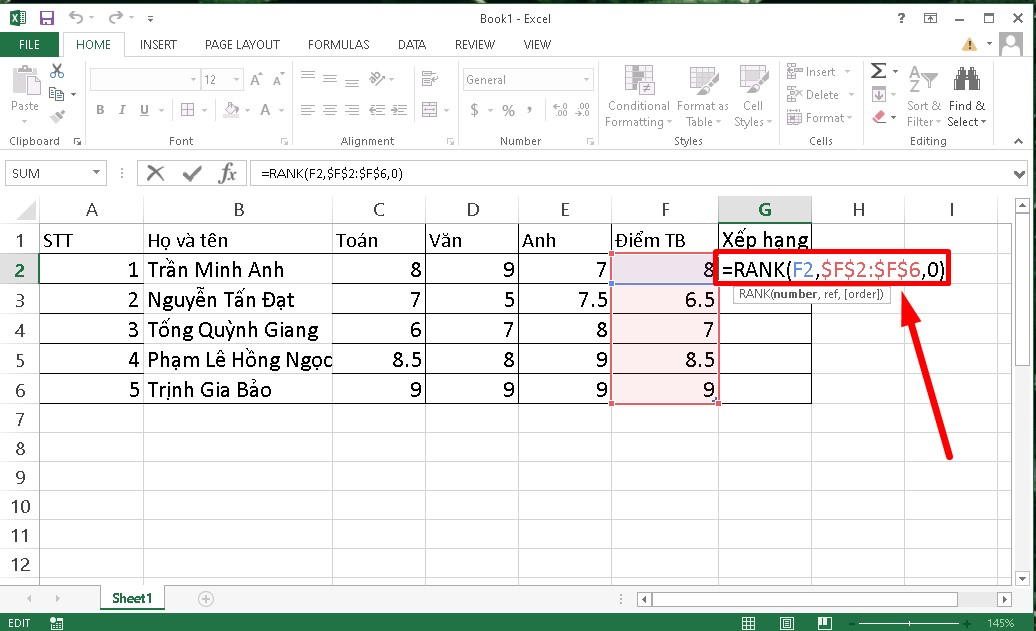In there:

• Number: The value you want to rate.
• Ref: List of numbers to sort
• Order: Order to be ranked, sort type (ascending or descending). If Order = 0 The rankings will be sorted in descending order. Still Order = 1 The rankings will be sorted in ascending order.

### Use the Rank function to rank the order from high to low

Step 1: In the cell located at the first position of the column to be ranked > You proceed to enter the formula =RANK(F2,\$F\$2:\$F\$5,0).Step 2: After entering the function into the rating field, you Press Enter for the system to return the results for you. At this time, the table will return a result of 3, ie Tran Minh Anh has a rank of 3.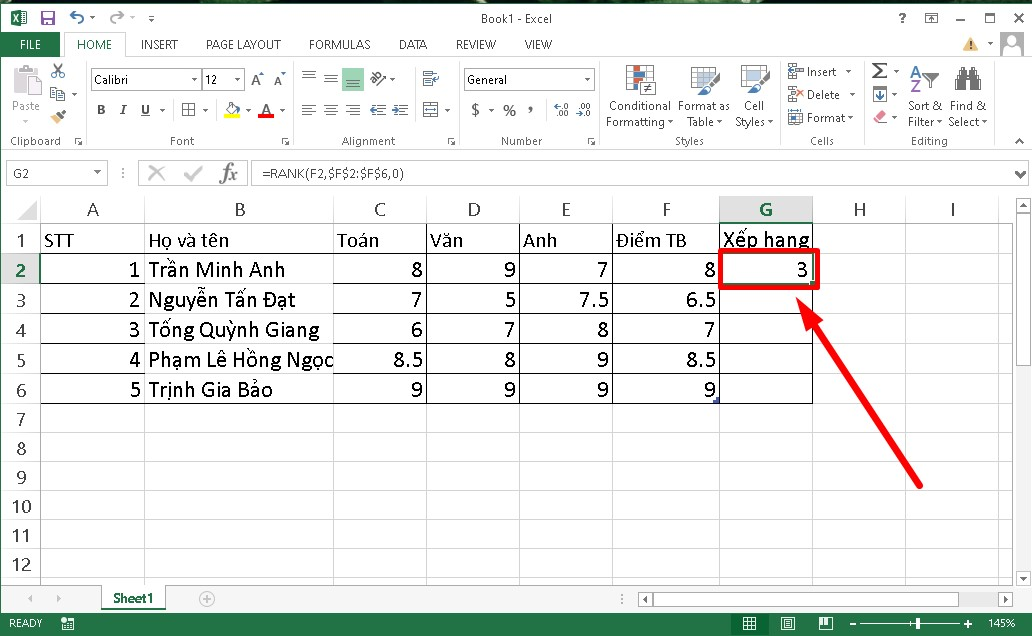Step 3: Next, to display the entire ranking of the next students proceed to move the mouse pointer to the lower right corner of the location containing the result > At this point, a plus sign appears, you hold the mouse and drag down until the list is finished.

Step 4: Result after execution: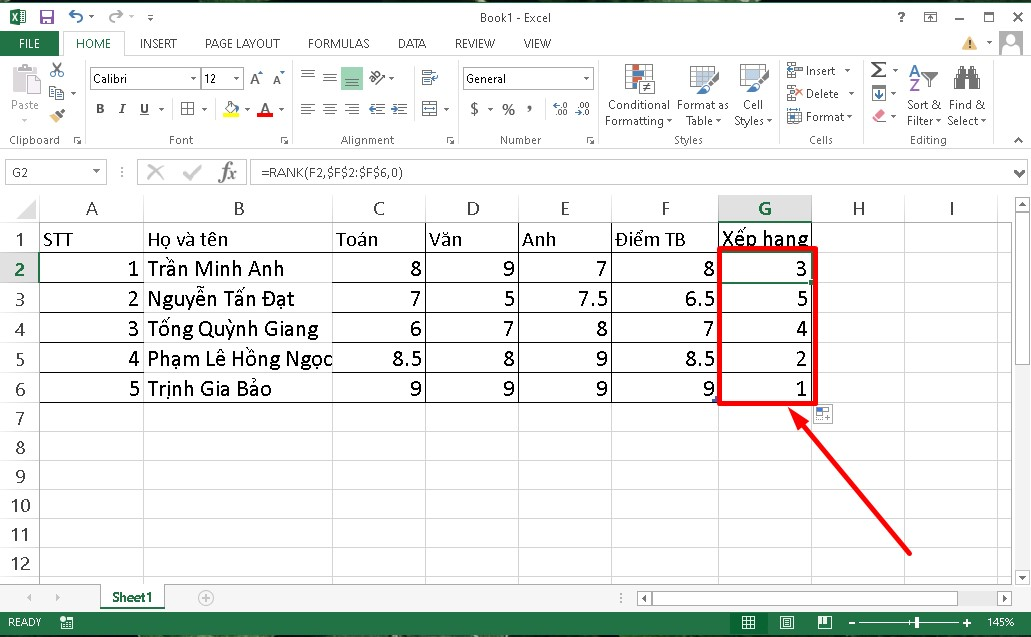• In case the data table contains duplicate numbers, when performing the ranking, they will tend to have the same rank and affect the next rankings.
• Such as, in the data table below contains the number 8.666667 If they appear twice, they will both be ranked 1st. Therefore, when the next rank is 2 for a score of 8 will be affected and will change the rank to 4th.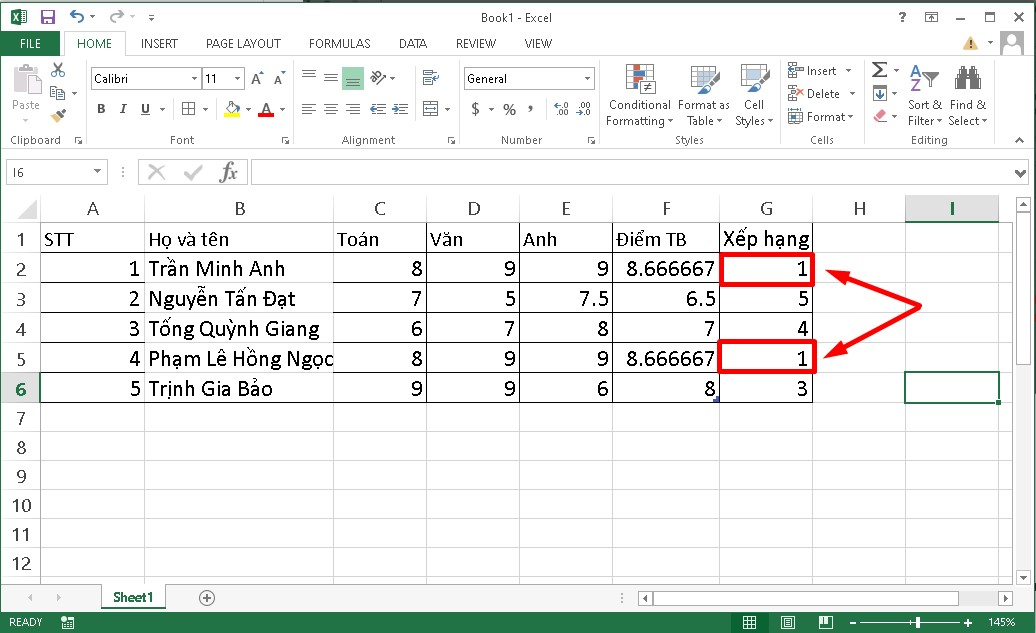### Use the Rank function in Excel to rank from low to high.

Note: In the case of ranking in order from low to high, the ranking to be calculated will be reversed. That is, the higher the score in the table will be in the lowest position and vice versa. When using the function counting from low to high, then order = 1.

Step 1: Proceed to enter the formula into the cell to be ranked first in the table using the following function: =RANK(B2,\$B\$2:\$B\$5,1).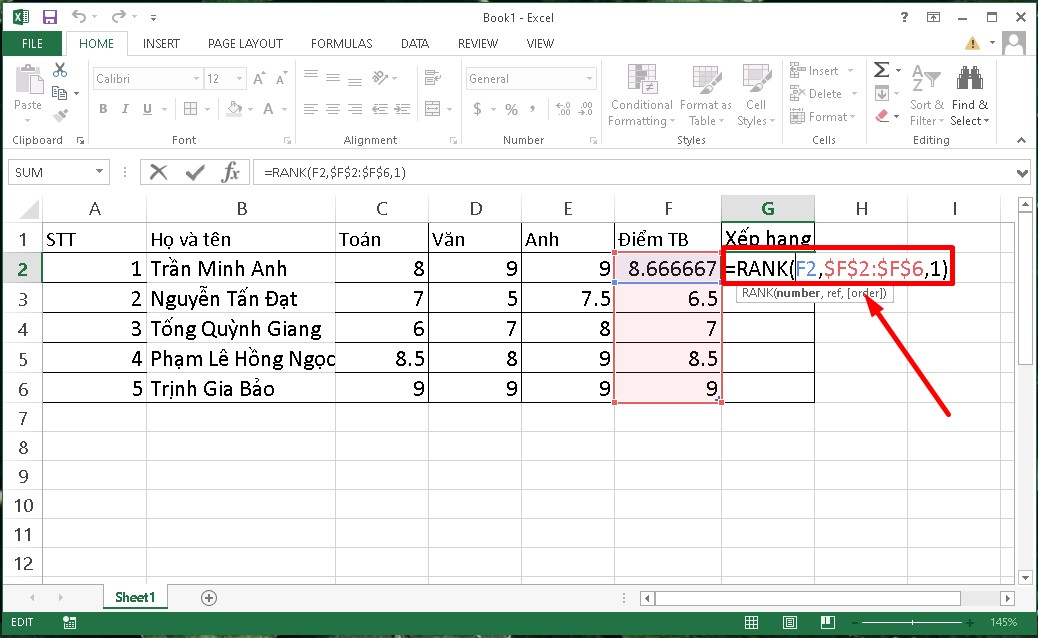Step 2: Press Enter and the system will return the results to the table. When implementing this formula, Tran Minh Anh will be ranked 4th.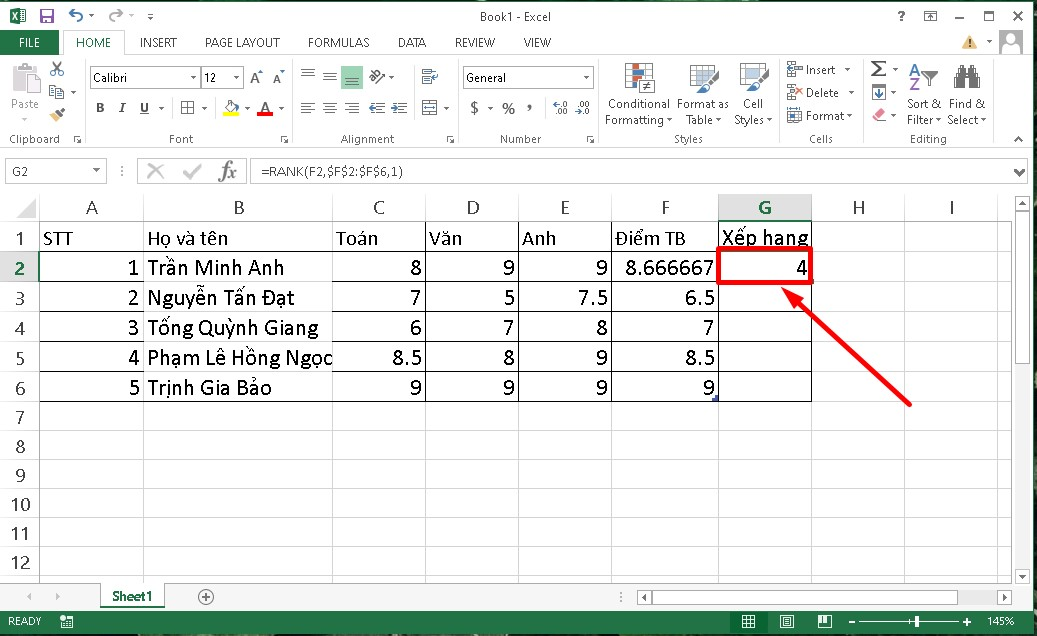Step 3: Next, to display the rank for the entire list, you Place the mouse pointer in the lower right corner of the data cell containing the first result > Hold down the mouse button until the end of the list need rating.

The results after doing so are as follows: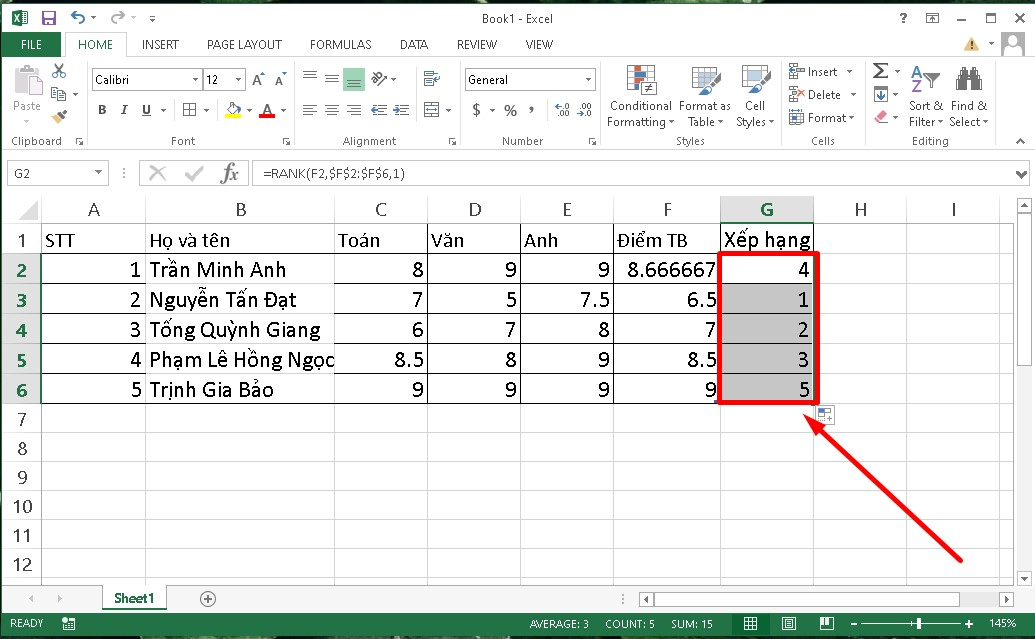## Ending

Above, Sforum showed you how to use the Rank function in Excel quickly and simply. Hopefully with the short article that we share will be useful to you in the process. Good luck.

Published